# Values

Retrieve individual point data on any curve in the active window. When a point is selected, the point data is displayed on the panel.

From the Complex Chart ribbon, click the Values tool.

When a point is selected, the point data is displayed on the panel. There are three ways to retrieve point data from a curve. A point can be picked from a curve, located using the Find Point controls, or selected from the point list.

## Find Point Controls

The range boundaries can also be picked using the Find point controls.
Click
To Find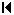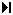the left-most or right-most end point.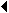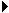the next point to the left or right.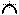global maximum of the curve.the next local maximum to the left or right.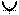the global minimum of the curve.the next local minimum to the left or right.
Next Curve
the corresponding point on the next curve.

## Point List

The point list displays the XY data points for the selected curve. The point number, the X-value, and the Y-value for each point is displayed in the list. Use the scroll bars to view the entire list. Click on a point in the list to display the point data.

## Point Data

The following data is displayed when a point is picked:
Curve

The name of the curve.

Point
The number of the data point.
X
The X value of the data point.
Y
The Y value of the data point.
Slope
An estimate of the slope of the curve at the selected point.
Time
The timestep of the data point (if available).

## Displaying Point Data

Under Show X as and Show Y as, you can choose from the following options to display the point data:
• Linear
• db10, to display data in decibels
• db20, to display data in decibels

Select Sync Coordinate Data with Axis Format to show coordinate info curve values in the same format (scientific, fixed) as used by the axis. See the Axes panel for more information on defining the axes.